# Chapter 18 Surface Area and Volume of Cuboid and Cube RD Sharma Solutions Exercise 18.1 Class 9 Maths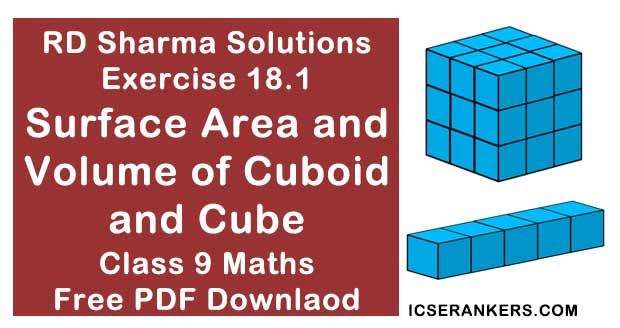Chapter Name RD Sharma Chapter 18 Surface Area and Volume of Cuboid and Cubes Exercise 18.1 Book Name RD Sharma Mathematics for Class 10 Other Exercises Exercise 18.2 Related Study NCERT Solutions for Class 10 Maths

### Exercise 18.1 Solutions

1. Find the lateral surface area and total surface area of a cuboid of length 80 cm, breadth 40cm and height 20 cm.

Solution

It is given that
Cuboid length = 80 cm = L
Breath = 40 cm  = b
Height = 20 cm  = h
WKT,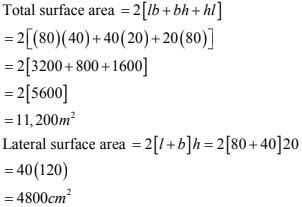2. Find the lateral surface area and total surface area of a cube of edge 10 cm.

Solution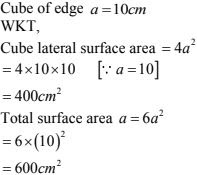3. Find the ratio of the total surface area and lateral surface area of a cube.

Solution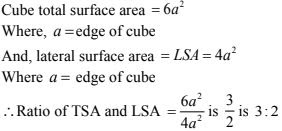4. Mary wants to decorate her Christmas tree. She wants to place the tree on a wooden block covered with coloured paper with picture of Santa Claus on it. She must know the exact quantity of paper to buy for this purpose. If the box has length, breadth and height as 80 cm, 40 cm and 20 cm respectively. How many square sheets of paper of side 40 cm would she require?

Solution

Given that mary wants to paste the paper on the outer surface of the box; The quantity of the paper required would be equal to the surface area of the box which is of the shape of cuboid. The dimension of the box are
Length (l) = 80 cm Breath (b) = 40 cm and height (h) = 20 cm
The surface area of thee box = 2[lb + bh + hl]
= 2[80(40) + 40(20) + 20(80)]5. The length, breadth and height of a room are 5 m, 4 m and 3 m respectively. Find the cost
of white washing the walls of the room and the ceiling at the rate of Rs. 7.50 m2 .
Solution
Total area to be washed = lb + 2(l + b)h
Where length (l) = 5 m
Breath (b) = 4 m
Height (h) = 3 m
∴ Total area to be white washed = (5 × 4) + 2(5 + 4) × 3 = 20 + 54 = 74 m2 .
Now,
Cost of white washing 1 m2 is Rs. 7.50
∴ Cost of white washing 74m2 is Rs. (74 × 7.50) = Rs. 555

6. Three equal cubes are placed adjacently in a row. Find the ratio of total surface area of the
new cuboid to that of the sum of the surface areas of the three cubes.
Solution
Length of new cuboid = 3a
Height of new cuboid = a
The total surface area of new cuboid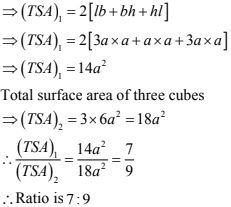7. A 4 cm cube is cut into 1 cm cubes. Calculate the total surface area of all the small cubes.
Solution
Edge of  cube = 4cm
Volume of 4 cm cube = (4 cm)3 = 64 cm2
Edge of cube  = 1 cm
Volume of 1cm cube = (1 cm)3 = 1 cm3
∴ Total number of small cubes  = 64 cm3 /1 cm3 = 64
∴ Total surface area of 64 cm all cubes
= 64 × 6 × (1 cm)2
= 384 cm2

8. The length of a hall is 18 m and the width 12 m. The sum of the areas of the floor and the flat roof is equal to the sum of the areas of the four walls. Find the height of the hall.
Solution
Length of the hall  = 18 m
Width of hall  = 12 m
Now given,
Area of the floor and the flat roof = Sum of the areas of four walls.
⇒ 2lb = 2lh + 2bh
⇒ lb = lh + bh
⇒ h = lb/(l + b) = (18 ×12)/(18 + 12) = 216/30 = 7.2 m

9. Hameed has built a cubical water tank with lid for his house, with each other edge 1 .5 m long. He gets the outer surface of the tank excluding the base, covered with square tiles of side 25 cm. Find how much he would spend for the tiles, if the cost of tiles is Rs. 360 per dozen.
Solution
Given that
Hameed is giving 5 outer faces of the tank covered with tiles he would need to know the surface area of the tank, to decide on the number of tiles required.
Edge of the cubic tank  = 1.5 m = 150 cm = a
So, surface area of tank  = 5 × 150 × 150 cm2
Area of each square tile = surface area of tank/area of each title
= (5 × 150 ×150)/(25 ×25) = 180
Cost of 1 dozen tiles i.e., cost of 12 tiles = Rs. 360
Therefore, cost of 12 balls tiles = Rs. 360
∴ Cost of one tile  = 360/12 = Rs.  30
∴ The cost of 180 tiles = 180 × Rs. 30 = Rs. 5,400

10. Each edge of a cube is increased by 50% . Find the percentage increase in the surface area of the cube.
Solution
Let d be the edge of the cube
∴ surface area of cube = 6 × a2
i.e, S1 = 6a2
According to problem when edge increased by 50% then the new edge becomes
= a + 50/100 × a
= 3a/2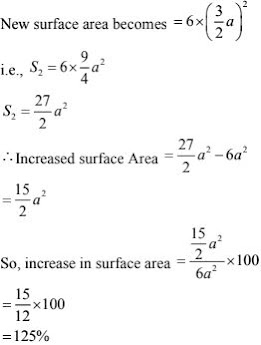11. The dimensions of a rectangular box are in the ratio of 2 : 3 : 4 and the difference between
the cost of covering it with sheet of paper at the rates of Rs. 8 and Rs. 9.50 per m2  is Rs. 1248. Find the dimensions of the box.
Solution
Let the ratio be x
∴ Length = 2x
Breath = 3x
Height  = 4x
∴ Total surface area = 2[lb + bh + hl]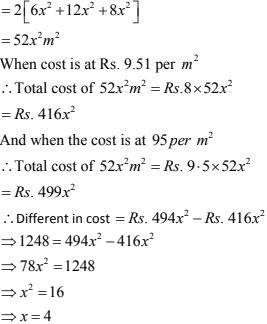12. A closed iron tank 12 m long, 9 m wide and 4 m deep is to be made. Determine the cost of
iron sheet used at the rate of Rs. 5 per metre sheet, sheet being 2 m wide.
Solution
Given length  = 12m, Breadth  = 9m and Height  = 4m.
Total surface area of tank = 2(lb + bh + hl)
= 2[12×9 + 9× 4 + 12 × 4]
= 2[108 + 36 + 48]
= 384 m2
Now length of iron sheet = 384/width of iron sheet
= 384/2 = 192 m.
Cost of iron sheet = length of sheet × cost rate
= 192 × 5 = Rs. 960.

13. Ravish wanted to make a temporary shelter for his car by making a box-like structure with
tarpaulin that covers all the four sides and the top of the car ( with the front face as a flap which can be rolled up). Assuming that the stitching margins are very small, and therefore negligible, how much tarpaulin would be required to make the shelter of height 2.5 m with base dimensions 4 m × 3m?
Solution
Given that
Shelter length  = 4 m
Height = 2.5 m
The tarpaulin will be required for four sides of the shelter
Area of tarpaulin in required = 2[lb + bh + hl]
= 2[2(4) × 2.5 + (3×2.5)] + 4 ×3] m2
= [2(10 + 7.5) + 12]m2
= 47m2

14. An open box is made of wood 3cm thick. Its external length, breadth and height are 1.48 m , 1.16m and 8.3 m. Find the cost of painting the inner surface of Rs 50 per sq. metre.
Solution
Given
Length = 1.48m = 148 cm.
Breath = 1.16 m = 116 cm
Height = 8.3 m = 83 cm
Thickness of wood = 3 cm
∴ inner dimensions:
Length (148 - 2 ×3) cm = 142 cm
Breadth (116 - 2 × 3) cm = 110cm
Height  = (83 - 3) cm = 80 cm.
Inner surface area = 2(l + b) + lb
= 2[142 + 110]80 + 142×110 cm2
= 2[252 × 80] + 142 × 110 cm2  = 55, 940 cm2 = 559.40m2
Hence, cost of painting inner surface area
= 55940 × Rs. 50
= Rs. 279.70

15. The cost of preparing the walls of a room 12 m long at the rate of Rs. 1.35 per square metre is Rs. 340.20 and the cost of matting the floor at 85 paise per square metre is Rs. 91.80. Find the height of the room.
Solution
Given that
Length of room = 12 m.
Let height of room be 'h' m.
Area of 4 walls = 2(l + b) × h
According to question
⇒ 2(l + b) × h ×1.35 = 340.20
⇒ 2(12 + b) × h ×1.35 = 340.20
⇒ (12 + b) ×h = 170.10/1.35 = 126 ...(1)
Also area of floor = l × b
∴ l × b × 0.85 = 91.80
⇒ 12 × b × 0.85 = 91.80
⇒ b = 9 m ...(2)
Substituting b = 9 m in equation (1)
⇒ (12 + 9) × h = 126
⇒ h = 6 m

16. The dimensions of a room are 12.5 m by  9m by  7m. There are 2 doors and 4 windows in
the room; each door measures 2.5 m by 1 .2 m and each window 1 .5 m by I m. Find the cost of painting the walls at Rs. 3.50 per square metre.
Solution
Given length of room = 12.5 m
Breadth of room = 9 m
Height of room = 7 m
∴ Total surface area of  4 walls
= 2(l + b) × h
= 2(12.5 + 9) × 7
= 301 m2
Area of 2 doors = 2[2.5 ×1.2] = 6m2
Area to be painted on 4 walls
= 301 - (6 + 6)
= 301 - 12 = 289 m2
∴ cost of painting  = 289 × 3.50 = Rs. 1011.5 .

17. The length and breadth of a hall are in the ratio 4: 3 and its height is 5.5 metres. The cost of
decorating its walls (including doors and windows) at Rs. 6.60 per square metre is Rs. 5082. Find the length and breadth of the room.
Solution
Let the length be 4x and breadth be 3x
Height  = 5.5 m  [given]
Now it is given that cost of decorating 4 walls at the rate of Rs. 6.601m2  is Rs. 5082.
⇒ Area of four walls × rate = total cost of painting
2(l + b) × h × 6.60 = 5082
2(4x + 3x) ×5.5 × 6.60 = 5082
⇒ 7x = 5082/(5.5 × 2.6 × 2)
⇒ 7x = 10
⇒ x = 10
Length = 4x = 4× 10 = 40m
Breadth = 3x = 3 ×10 = 30 m

18. A wooden bookshelf has external dimensions as follows: Height = 110 cm, Depth = 25 cm,
Breadth = 85 cm (See Fig. 18.5). The thickness of the plank is 5 cm everywhere. The external
faces are to be polished and the inner faces are to be painted. If the rate of polishing is 20 paise per cm2  and the rate of painting is 10 paise per cm2 . Find the total expenses required for polishing and painting the surface of the bookshelf.
Solution
External length of book shelf  = 85 cm  = l
Height  = 110 cm.
External surface area of shelf while leaving front face of shelf
= lh + 2(lb + bh)
= [85 × 110 + 21(85 × 25 + 25 ×110)] cm2
= 19100 cm2
Area of front face = (85 × 110 - 75 ×100 + 2(75 ×5)) cm2
= 1850 + 750 cm2
= 2600 cm2
Area to be polished  = 19100 + 2600 cm2
= 21700 cm2
Cost of polishing 1 cm2 area = Rs. 0.20
Cost of polishing 21700 cm2 area = Rs. [21700 × 0.20]
= Rs. 4340
Now, length (l), breath (b), height (h) of each row of book shelf is 75 cm, 20cm and 30 cm
= (110 - 20)/3 respectively.
Area to be painted in row = 2(l + h)b + lh
= [2(75 + 30)20 + 75 ×30] cm2
= (4200 + 2250) cm2 = 6450 cm2
Area to be painted in 3 rows  = (3 × 6450) cm2
= 19350 cm2
Cost of painting 1 cm2 area = Rs. 0.10
Cost of painting 19350 area = Rs. (19350 ×0.10) - Rs. 1935
Total expense required for polishing and painting the surface of the bookshelf
= Rs.(4340 + 1935) = Rs. 6275.

19. The paint in a certain container is sufficient to paint on area equal to 9.375 m2 . How many bricks of dimension 22.5 cm × 10 cm  × 7.5 cm can be painted out of this container ?
Solution
We know that
Total surface area of one brick = 2(lb + bh + hl)
= 2[22.5 × 10 + 10 ×7.5 + 22.5 × 7.5] cm2
= 2[468.75]cm2
= 937.5 cm2
Let n number of bricks be painted by the container
Area of brick = 937. 50 cm2
Area that can be painted in the container
= 93755 m2 = 93750 cm2
93750 = 937.5 n
n = 100
Thus, 100 bricks can be painted out by the container.